# Continuing on working on my mouse moves in DrawSketchHandlerLineSet

Ok… So I thinking I’m approaching a point where the rubber hits the road…. Or  not… So… What I’m attempting to do is to add an arc to the polyline mode.

I came up with a sketch for solving the polyline arc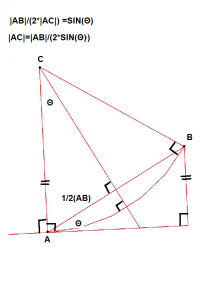So basically A would be the first mouse click and B would be the second mouse click.

So what I need to do is to solve for C which would be the center of the arc.

Goal:
The goal in this sub-project is to determine  the vector position of the center of the Arc “C”.  Once  worked out, I’m on easy street (in this sub-project anyway) for  drawing the Arc in the mouse movement  since the code has  already been worked out

Figuring out the scalar value of VC what I need to do is to figure out the vector position of C

“A” would be the end point of the previous element and the starting point of the Arc.  It would make sense that if we’re drawing a polyline that the Arc should be tangent to the previous element.

That begs the questions… Is there a previous element. Looking at the code, I believe that question could be answered by getHighestCurveIndex().  The question, is that does this return if there is no prior curve?

This function is defined in:
class SketcherExport SketchObject : public Part::Part2DObject
as:
int getHighestCurveIndex(void) const { return Geometry.getSize() – 1; }

Stepping into this:

int DrawSketchHandler::getHighestCurveIndex(void)
{
return sketchgui->getSketchObject()->getHighestCurveIndex();
}
—-
Sketcher::SketchObject *ViewProviderSketch::getSketchObject(void) const
{
return dynamic_cast<Sketcher::SketchObject *>(pcObject);
}

—-

in SketchObject

—-

int getHighestCurveIndex(void) const { return Geometry.getSize() – 1; }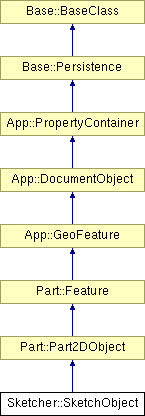int PropertyGeometryList::getSize(void) const
{
return static_cast<int>(_lValueList.size());
}

where Geometry is defined as:

/// Property
Part    ::PropertyGeometryList   Geometry;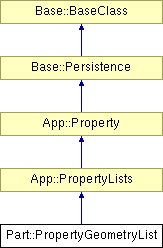int PropertyGeometryList::getSize ( void ) const` [virtual]`

Implements App::PropertyLists.

Definition at line 75 of file PropertyGeometryList.cpp.

int PropertyGeometryList::getSize(void) const
{
return static_cast<int>(_lValueList.size());
}

So… after all that if getHighestCurveIndex returns -1 then there is no preset curve and I need to fake a tangent..
Otherwise I should pick tangent to the last element…
So  I think I just need to add a few  vectors:
Base::Vector2D centerPoint,tangent,distance;

This is cool check out the Vector2D member functions.  There’s a bunch I can use here.

 float GetAngle (const Vector2D &rclVect) const float Length (void) const void Normalize (void) float operator* (const Vector2D &rclVct) const Vector2D operator+ (const Vector2D &rclVct) const Vector2D operator- (const Vector2D &rclVct) const Vector2D & operator= (const Vector2D &rclVct) bool operator== (const Vector2D &rclVct) const void ProjToLine (const Vector2D &rclPt, const Vector2D &rclLine) void Scale (float fS) void Set (float fPX, float fPY) Vector2D (const Vector2D &rclVct) Vector2D (double x, double y) Vector2D (float x, float y) Vector2D (void)

One thing that I still need to do is to execute a vector cross product. I was have discussion of how to do this on the #freecad IRC and one had showed how to do this in python:https://gist.github.com/2297556

def getTangent(edge):
“Returns a tangent vector from an edge in FreeCAD”
if isinstance(edge.Curve,Part.Line):
vec = edge.Vertexes[-1].Point.sub(edge.Vertexes.Point)
elif isinstance(edge.Curve,Part.Circle):
v1 = edge.Vertexes[-1].sub(edge.Curve.Center)
v2 = edge.Curve.Axis
vec = v1.cross(v2)
else:
print “not supported”
vec = None
return vec
So the question became where does python wrap around the c++ code?   The suggestion came to look at /home/jonas/freecad/free-cad/src/Base/VectorPy.cpp
What was really curious was that this file exists but doesn’t exist as a QT project file, looking inside the file explains why.
// This file is generated by src/Tools/generateTemaplates/templateClassPyExport.py out of the .XML file
// Every change you make here get lost at the next full rebuild!
// This File is normaly build as an include in VectorPyImp.cpp! Its not intended to be in a project!
Looking at Base::VectorPy
PyObject*  VectorPy::cross(PyObject *args)
{
PyObject *obj;
if (!PyArg_ParseTuple(args, “O!”, &(VectorPy::Type), &obj))
return 0;VectorPy* vec = static_cast<VectorPy*>(obj);VectorPy::PointerType this_ptr = reinterpret_cast<VectorPy::PointerType>(_pcTwinPointer);
VectorPy::PointerType vect_ptr = reinterpret_cast<VectorPy::PointerType>(vec->_pcTwinPointer);Base::Vector3d v = (*this_ptr) % (*vect_ptr);
return new VectorPy(v);
}
and?
PyObject*  VectorPy::sub(PyObject *args)
{
PyObject *obj;
if (!PyArg_ParseTuple(args, “O!”, &(VectorPy::Type), &obj))
return 0;VectorPy* vec = static_cast<VectorPy*>(obj);VectorPy::PointerType this_ptr = reinterpret_cast<VectorPy::PointerType>(_pcTwinPointer);
VectorPy::PointerType vect_ptr = reinterpret_cast<VectorPy::PointerType>(vec->_pcTwinPointer);Base::Vector3d v = (*this_ptr) – (*vect_ptr);
return new VectorPy(v);
}
so sub is vector subtraction… duh….
So… I managed to get rid of seg-faults in the mouse move and I’m starting to get a polyline arc… Sort of
This is amusing to me. I copied the code from DrawSketchHandlerArc and I neglected to populate the variables rx and ry
So I fixed that and thought for the mouse move, add a couple of more lines which would would go from last point to center, to first point… and here is the result.
That makes things a little more interesting.
The one radius line on this arc is supposed to be perpendicular to the line… Clear its not.. Something is obviously not correct..
Oh… this might be it… duhhh:
tangent.Set(lineSeg->getEndPoint().x-lineSeg->getStartPoint().x,
lineSeg->getEndPoint().x-lineSeg->getStartPoint().y,
0.0);
Ok.. now we have this.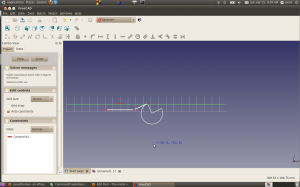It’s not up in this picture but I need to fix if the center of the arc is above the line or below the line.
Also not really represented in this picture, I think something is screwed up in my radius length calculation of the vector from the endpoint of the previous line.
Double duhh..
s/b
Ok… this is looking somewhat better but the arc should be on the cursor..
Oh… Another silly bug.
I copied the code for the arc from DrawSketchHandlerArc whose behavior is different.
The critter lies here:
arcAngle = abs(angle1-arcAngle) < abs(angle2-arcAngle) ? angle1 : angle2;
arcAngle must be initially calculated in DrawSketchHandlerArc
Success
So… I got this working (barely on the mouse move but..at least its a start)
I need to get the center to flip when the center goes above the line.
4/17 update
I’m widdling down ther issues, but now I have two issues that I need to work out.
1) When arc gets to 180 degrees the arc reverses.
2) The curve is not breaking to the right

So.. I think issue 1) is easy…

and

http://www.cplusplus.com/reference/clibrary/cmath/atan2/
Atan2 returns Principal arc tangent of y/x, in the interval [-pi,+pi] radians.
What I need to wind up with is 0 to 2PI if I’m going to the left CCW and 0 to -2PI if I’m going CCW.
So.. I need to factor direction when I’m calculating the angle… (More on this later)
I had this vector reference bookmarked at work.. It’s a nice site… This really belongs in my useful links, but good for know

http://chortle.ccsu.edu/VectorLessons/vch09/vch09_6.html

4-19 update…Getting tanalizing closer… Stupid logic errors that I almost have worked out for the CCW polyline arc.  I still done have the mousedown event written to draw the arc, so I revert to line (key up on the “A” to test the next case.  Here’s where it’s bombing.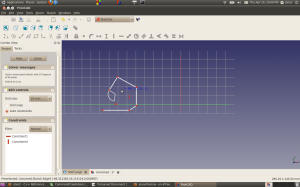4-21 update. Yeah… Arcs to draw out properly CCW condition. I’m going to get the  the code to respond to a hard set clockwise before start working on the CCW/CW selector.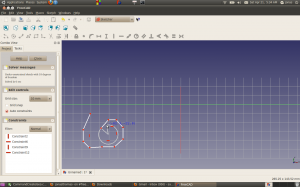This is the little section of code(Which works at the moment) that caused me such pain, no big deal..

(Just in case I need to get back to this point

// if ((kVec.z ==-1) ){//ccw
if (startAngle<=0 && finishAngle>=0  )
arcAngle = finishAngle – startAngle ;
else if (startAngle>=0  && finishAngle<=0 )
arcAngle = abs(finishAngle+ 2 * M_PI – startAngle);
else if(( startAngle<0 && finishAngle<0 )|| (startAngle>0 && finishAngle>0 )){//somewhere in the 3 or 4 quandrant
if (finishAngle>startAngle){
arcAngle =finishAngle-startAngle;

}
else{
arcAngle =finishAngle-startAngle+ 2* M_PI;
}

}
else
arcAngle = abs(finishAngle – startAngle);
//  }
// else
//  {

//  }
—–
Ok… I think I got the arcs to draw out properly for both ccw and cw conditions. Here’s the magic:

if ((kVec.z ==-1) ){//ccw
if (startAngle<=0 && finishAngle>=0  )
arcAngle = finishAngle – startAngle ;
else if (startAngle>=0  && finishAngle<=0 )
arcAngle = abs(finishAngle+ 2 * M_PI – startAngle);
else if(( startAngle<0 && finishAngle<0 )|| (startAngle>0 && finishAngle>0 )){//BOTH START AND FINISH EITHER UPPER OR LOWER QUANDRANTS
if (finishAngle>startAngle){
arcAngle =finishAngle-startAngle;

}
else{
arcAngle =finishAngle-startAngle+ 2* M_PI;
}

}
else
arcAngle = abs(finishAngle – startAngle);//Don’t think this point is ever reached
}
else{//cw rotation
if (startAngle<=0 && finishAngle>=0  )
arcAngle = abs(finishAngle – startAngle-2 *M_PI) ;
else if (startAngle>=0  && finishAngle<=0 )
arcAngle = abs( -finishAngle + startAngle);
else if(( startAngle<0 && finishAngle<0 )|| (startAngle>0 && finishAngle>0 )){//BOTH START AND FINISH EITHER UPPER OR LOWER QUANDRANTS
if (finishAngle>startAngle){

arcAngle =abs(finishAngle-startAngle- 2* M_PI);

}
else{
arcAngle =abs(finishAngle-startAngle);

}

}
else
arcAngle = abs(finishAngle – startAngle);
}

rx = EditCurve.fX – CenterPoint.fX;
ry = EditCurve.fY – CenterPoint.fY;
for (int i=1; i <= 29; i++) {
float angle = i*arcAngle/29.0;
float dx,dy;
if (kVec.z ==-1){
dx = rx * cos(angle) – ry * sin(angle);
dy = rx * sin(angle) + ry * cos(angle);
}
else{
dx = rx * cos(-angle) – ry * sin(-angle);
dy = rx * sin(-angle) + ry * cos(-angle);
}

EditCurve[i] = Base::Vector2D(CenterPoint.fX + dx, CenterPoint.fY + dy);
}
EditCurve=CenterPoint;
EditCurve= EditCurve;

sketchgui->drawEdit(EditCurve);
if (seekAutoConstraint(sugConstr3, onSketchPos, Base::Vector2D(0.f,0.f))) {
renderSuggestConstraintsCursor(sugConstr3);
return;
}
break;
}
applyCursor();

}

So… Unless I missed something as far as the arc logic in mouse moves… It’s done…. Now on to setting cw and ccw

This entry was posted in Uncategorized. Bookmark the permalink.